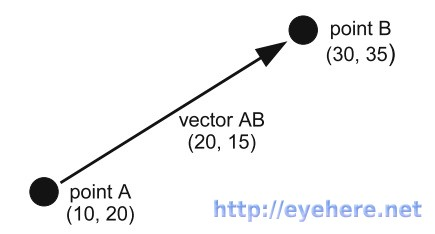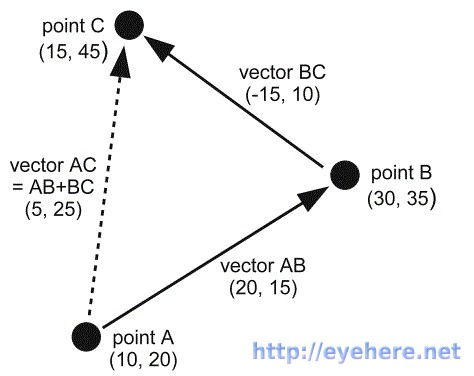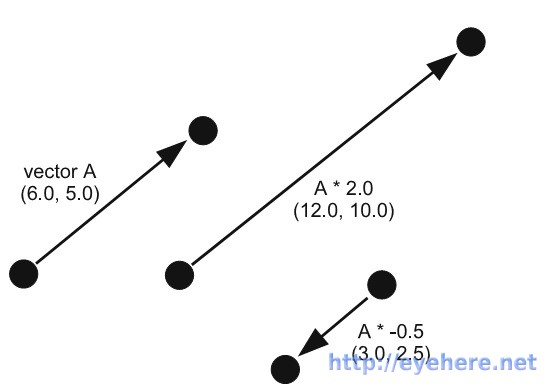如何用Pygame写游戏（九）“一方通行”凭借向量的力量成为了学园第一能力者，我们是否也应该好好学习向量？

引入向量class Vector2(object):    def __init__(self, x=0.0, y=0.0):        self.x = x        self.y = y    def __str__(self):        return "(%s, %s)"%(self.x, self.y)     @classmethod    def from_points(cls, P1, P2):        return cls( P2 – P1, P2 – P1 )#我们可以使用下面的方法来计算两个点之间的向量A = (10.0, 20.0)B = (30.0, 35.0)AB = Vector2.from_points(A, B)print AB

向量的大小

    def get_magnitude(self):        return math.sqrt( self.x**2 + self.y**2 )

单位向量

    def normalize(self):        magnitude = self.get_magnitude()        self.x /= magnitude        self.y /= magnitude

向量运算(20, 15) + (-15, 10) = (20-15, 15+10) = (5, 25)

    def __add__(self, rhs):        return Vector2(self.x + rhs.x, self.y + rhs.y)    def __sub__(self, rhs):        return Vector2(self.x - rhs.x, self.y - rhs.y)def __mul__(self, scalar):        return Vector2(self.x * scalar, self.y * scalar)    def __div__(self, scalar):        return Vector2(self.x / scalar, self.y / scalar)

更好的向量类

from gameobjects.vector2 import *A = (10.0, 20.0)B = (30.0, 35.0)AB = Vector2.from_points(A, B)print "Vector AB is", ABprint "AB * 2 is", AB * 2print "AB / 2 is", AB / 2print "AB + (–10, 5) is", AB + (–10, 5)print "Magnitude of AB is", AB.get_magnitude()print "AB normalized is", AB.get_normalized() # 结果是下面Vector AB is ( 20, 15 )AB * 2 is ( 40, 30 )AB / 2 is ( 10, 7.5 )AB + (-10, 5) is ( 10, 20 )Magnitude of AB is 25.0AB normalized is ( 0.8, 0.6 )

使用向量的游戏动画

background_image_filename = 'sushiplate.jpg'sprite_image_filename = 'fugu.png' import pygamefrom pygame.locals import *from sys import exitfrom gameobjects.vector2 import Vector2 pygame.init() screen = pygame.display.set_mode((640, 480), 0, 32) background = pygame.image.load(background_image_filename).convert()sprite = pygame.image.load(sprite_image_filename).convert_alpha() clock = pygame.time.Clock() position = Vector2(100.0, 100.0)heading = Vector2() while True:     for event in pygame.event.get():        if event.type == QUIT:            exit()     screen.blit(background, (0,0))    screen.blit(sprite, position)     time_passed = clock.tick()    time_passed_seconds = time_passed / 1000.0     # 参数前面加*意味着把列表或元组展开    destination = Vector2( *pygame.mouse.get_pos() ) - Vector2( *sprite.get_size() )/2    # 计算鱼儿当前位置到鼠标位置的向量    vector_to_mouse = Vector2.from_points(position, destination)    # 向量规格化    vector_to_mouse.normalize()     # 这个heading可以看做是鱼的速度，但是由于这样的运算，鱼的速度就不断改变了    # 在没有到达鼠标时，加速运动，超过以后则减速。因而鱼会在鼠标附近晃动。    heading = heading + (vector_to_mouse * .6)         position += heading * time_passed_seconds    pygame.display.update()

动画总结

• 正如上一章所说，所谓动画，不过是在每一帧上，相对前一帧把精灵的坐标在加减一些而已；
• 使用时间来计算加减的量以在不同性能的计算机上获得一致的动画效果；
• 使用向量来计算运动的过程来减轻我们的劳动，在3D的情况下，简单的使用Vector3便可以了。

        </div>